LORENE
Lorene::Eos_CompOSE Class Reference

Equation of state for the CompOSE database. More...

#include <eos_compose.h>

Inheritance diagram for Lorene::Eos_CompOSE: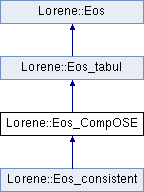## Public Member Functions

Eos_CompOSE (const string &files_path)
Constructor from CompOSE data. More...

Eos_CompOSE (const char *file_name)
Standard constructor. More...

virtual ~Eos_CompOSE ()
Destructor. More...

int get_format () const

virtual bool operator== (const Eos &) const
Comparison operator (egality) More...

virtual bool operator!= (const Eos &) const
Comparison operator (difference) More...

virtual int identify () const
Returns a number to identify the sub-classe of Eos the object belongs to. More...

virtual void sauve (FILE *) const
Save in a file. More...

const string & get_tablename () const

const Tblget_logh () const

double get_hmin () const
Returns minimal value of log-enthalpy in the table. More...

double get_hmax () const
Returns maximal value of log-enthalpy in the table. More...

virtual double nbar_ent_p (double ent, const Param *par=0x0) const
Computes the baryon density from the log-enthalpy. More...

virtual double ener_ent_p (double ent, const Param *par=0x0) const
Computes the total energy density from the log-enthalpy. More...

virtual double press_ent_p (double ent, const Param *par=0x0) const
Computes the pressure from the log-enthalpy. More...

virtual double der_nbar_ent_p (double ent, const Param *par=0x0) const
Computes the logarithmic derivative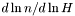from the log-enthalpy. More...

virtual double der_ener_ent_p (double ent, const Param *par=0x0) const
Computes the logarithmic derivative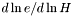from the log-enthalpy. More...

virtual double der_press_ent_p (double ent, const Param *par=0x0) const
Computes the logarithmic derivative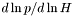from the log-enthalpy. More...

virtual double der_press_nbar_p (double ent, const Param *par=0x0) const
Computes the logarithmic derivative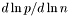from the log-enthalpy. More...

virtual double csound_square_ent_p (double, const Param *) const
Computes the sound speed squared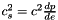from the enthapy with extra parameters (virtual function implemented in the derived classes). More...

const char * get_name () const
Returns the EOS name. More...

void set_name (const char *name_i)
Sets the EOS name. More...

Cmp nbar_ent (const Cmp &ent, int nzet, int l_min=0, Param *par=0x0) const
Computes the baryon density field from the log-enthalpy field and extra parameters. More...

Scalar nbar_ent (const Scalar &ent, int nzet, int l_min=0, Param *par=0x0) const
Computes the baryon density field from the log-enthalpy field and extra parameters. More...

Cmp ener_ent (const Cmp &ent, int nzet, int l_min=0, Param *par=0x0) const
Computes the total energy density from the log-enthalpy and extra parameters. More...

Scalar ener_ent (const Scalar &ent, int nzet, int l_min=0, Param *par=0x0) const
Computes the total energy density from the log-enthalpy and extra parameters. More...

Cmp press_ent (const Cmp &ent, int nzet, int l_min=0, Param *par=0x0) const
Computes the pressure from the log-enthalpy and extra parameters. More...

Scalar press_ent (const Scalar &ent, int nzet, int l_min=0, Param *par=0x0) const
Computes the pressure from the log-enthalpy and extra parameters. More...

Cmp der_nbar_ent (const Cmp &ent, int nzet, int l_min=0, Param *par=0x0) const
Computes the logarithmic derivativefrom the log-enthalpy and extra parameters. More...

Scalar der_nbar_ent (const Scalar &ent, int nzet, int l_min=0, Param *par=0x0) const
Computes the logarithmic derivativefrom the log-enthalpy and extra parameters. More...

Cmp der_ener_ent (const Cmp &ent, int nzet, int l_min=0, Param *par=0x0) const
Computes the logarithmic derivativefrom the log-enthalpy and extra parameters. More...

Scalar der_ener_ent (const Scalar &ent, int nzet, int l_min=0, Param *par=0x0) const
Computes the logarithmic derivativefrom the log-enthalpy and extra parameters. More...

Cmp der_press_ent (const Cmp &ent, int nzet, int l_min=0, Param *par=0x0) const
Computes the logarithmic derivativefrom the log-enthalpy and extra parameters. More...

Scalar der_press_ent (const Scalar &ent, int nzet, int l_min=0, Param *par=0x0) const
Computes the logarithmic derivativefrom the log-enthalpy and extra parameters. More...

Scalar csound_square_ent (const Scalar &ent, int nzet, int l_min=0, Param *par=0x0) const
Computes the sound speed squaredfrom the enthalpy with extra parameters. More...

## Static Public Member Functions

static Eoseos_from_file (FILE *)
Construction of an EOS from a binary file. More...

static Eoseos_from_file (ifstream &)
Construction of an EOS from a formatted file. More...

## Protected Member Functions

Eos_CompOSE (FILE *)
Constructor from a binary file (created by the function sauve(FILE*) ). More...

Eos_CompOSE (ifstream &)
Constructor from a formatted file. More...

Reads the files containing the table and initializes in the arrays logh , logp and dlpsdlh (CompOSE format). More...

virtual ostream & operator>> (ostream &) const
Operator >> More...

Reads the file containing the table and initializes in the arrays logh , logp and dlpsdlh . More...

void calcule (const Cmp &thermo, int nzet, int l_min, double(Eos::*fait)(double, const Param *) const, Param *par, Cmp &resu) const
General computational method for Cmp 's. More...

void calcule (const Scalar &thermo, int nzet, int l_min, double(Eos::*fait)(double, const Param *) const, Param *par, Scalar &resu) const
General computational method for Scalar 's. More...

## Protected Attributes

int format
0 for standard (old) LORENE format, 1 for CompOSE format More...

string tablename
Name of the file containing the tabulated data. More...

string authors
Authors - reference for the table. More...

double hmin
Lower boundary of the enthalpy interval. More...

double hmax
Upper boundary of the enthalpy interval. More...

Tbllogh
Table of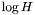. More...

Tbllogp
Table of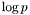. More...

Tbldlpsdlh
Table of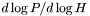. More...

Tbllognb
Table of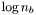. More...

Tbldlpsdlnb
Table of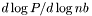. More...

Tbllog_cs2
Table of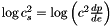. More...

double * press

double * nb

double * ro

char name 
EOS name. More...

## Private Member Functions

Eos_CompOSE (const Eos_CompOSE &)
Copy constructor (private to make Eos_CompOSE a non-copiable class) More...

## Friends

EosEos::eos_from_file (FILE *)
The construction functions from a file. More...

EosEos::eos_from_file (ifstream &)

## Detailed Description

Equation of state for the CompOSE database.

General tabulated EOS, reading a table passed as an argument to the constructor. When built with Eos::eos_from_file(), the file must be composed of the following lines:

17    Type of the EOS
1   0: standard format  1: CompOSE format
Tabulated EoS
/full/path/to/the/eos/table/name_of_the_table 

On the second line '0' means that the table has the standard LORENE format for tabulated EoSs (see class Eos_tabul for details). '1' means that the files from the CompOSE database are used and that the 'name_of_the_table' should be without suffix: e.g. great_eos would stand for files great_eos.nb and great_eos.thermo (see CompOSE documentation).

Definition at line 93 of file eos_compose.h.

## ◆ Eos_CompOSE() [1/5]

 Lorene::Eos_CompOSE::Eos_CompOSE ( const string & files_path )

Constructor from CompOSE data.

Parameters
 files_path Absolute name (including path), but without extensions of the CompOSE data, e.g. /home/foo/eos/my_eos standing for files my_eos.nb and my_eos.thermo as dowloaded from the CompOSE server.

Definition at line 135 of file eos_compose.C.

## ◆ Eos_CompOSE() [2/5]

 Lorene::Eos_CompOSE::Eos_CompOSE ( const char * file_name )

Standard constructor.

Parameters
 file_name Absolute name (including path) containing the EOS file. This file should contain a header with the following first lines: # Comments ... # Name of the authors / reference # Comments ... # Comments ... # Comments XXX <-- Number of lines # # index n_B [fm^{-3}] rho [g/cm^3] p [dyn/cm^2] #  where 'XXX' is the number of following lines of the EoS, each line containing an index (integer), baryon density, energy total density and pressure in the units given above.

Definition at line 102 of file eos_compose.C.

References Lorene::Eos_tabul::tablename.

## ◆ Eos_CompOSE() [3/5]

 Lorene::Eos_CompOSE::Eos_CompOSE ( FILE * fich )
protected

Constructor from a binary file (created by the function sauve(FILE*) ).

This constructor is protected because any EOS construction from a binary file must be done via the function Eos::eos_from_file(FILE*) .

Definition at line 110 of file eos_compose.C.

## ◆ Eos_CompOSE() [4/5]

 Lorene::Eos_CompOSE::Eos_CompOSE ( ifstream & fich )
protected

Constructor from a formatted file.

This constructor is protected because any EOS construction from a formatted file must be done via the function Eos::eos_from_file(ifstream& ) .

Definition at line 129 of file eos_compose.C.

## ◆ Eos_CompOSE() [5/5]

 Lorene::Eos_CompOSE::Eos_CompOSE ( const Eos_CompOSE & )
private

Copy constructor (private to make Eos_CompOSE a non-copiable class)

## ◆ ~Eos_CompOSE()

 Lorene::Eos_CompOSE::~Eos_CompOSE ( )
virtual

Destructor.

Definition at line 288 of file eos_compose.C.

## ◆ calcule() [1/2]

 void Lorene::Eos::calcule ( const Cmp & thermo, int nzet, int l_min, double(Eos::*)(double, const Param *) const fait, Param * par, Cmp & resu ) const
protectedinherited

General computational method for Cmp 's.

Parameters
 thermo [input] thermodynamical quantity (for instance the enthalpy field)from which the thermodynamical quantity resu is to be computed. nzet [input] number of domains where resu is to be computed. l_min [input] index of the innermost domain is which resu is to be computed [default value: 0]; resu is computed only in domains whose indices are in [l_min,l_min+nzet-1] . In the other domains, it is set to zero. fait [input] pointer on the member function of class Eos which performs the pointwise calculation. par possible extra parameters of the EOS resu [output] result of the computation.

Definition at line 213 of file eos.C.

References Lorene::Cmp::get_etat().

## ◆ calcule() [2/2]

 void Lorene::Eos::calcule ( const Scalar & thermo, int nzet, int l_min, double(Eos::*)(double, const Param *) const fait, Param * par, Scalar & resu ) const
protectedinherited

General computational method for Scalar 's.

Parameters
 thermo [input] thermodynamical quantity (for instance the enthalpy field)from which the thermodynamical quantity resu is to be computed. nzet [input] number of domains where resu is to be computed. l_min [input] index of the innermost domain is which resu is to be computed [default value: 0]; resu is computed only in domains whose indices are in [l_min,l_min+nzet-1] . In the other domains, it is set to zero. fait [input] pointer on the member function of class Eos which performs the pointwise calculation. par possible extra parameters of the EOS resu [output] result of the computation.

Definition at line 285 of file eos.C.

References Lorene::Scalar::get_etat().

## ◆ csound_square_ent()

 Scalar Lorene::Eos::csound_square_ent ( const Scalar & ent, int nzet, int l_min = 0, Param * par = 0x0 ) const
inherited

Computes the sound speed squaredfrom the enthalpy with extra parameters.

Parameters
 ent [input, unit: c^2] enthalpy nzet number of domains where the derivative dln(e)/dln(H) is to be computed. l_min index of the innermost domain is which the coefficient dln(n)/dln(H) is to be computed [default value: 0]; the derivative dln(e)/dln(H) is computed only in domains whose indices are in [l_min,l_min+nzet-1] . In the other domains, it is set to zero. par possible extra parameters of the EOS
Returns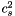[unit: c^2]

Definition at line 499 of file eos.C.

## ◆ csound_square_ent_p()

 double Lorene::Eos_tabul::csound_square_ent_p ( double ent, const Param * ) const
virtualinherited

Computes the sound speed squaredfrom the enthapy with extra parameters (virtual function implemented in the derived classes).

Parameters
 ent [input, unit: c^2] enthalpy par possible extra parameters of the EOS
Returns[unit: c^2]

Implements Lorene::Eos.

Reimplemented in Lorene::Eos_consistent.

Definition at line 599 of file eos_tabul.C.

## ◆ der_ener_ent() [1/2]

 Cmp Lorene::Eos::der_ener_ent ( const Cmp & ent, int nzet, int l_min = 0, Param * par = 0x0 ) const
inherited

Computes the logarithmic derivativefrom the log-enthalpy and extra parameters.

Parameters
 ent [input, unit: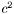] log-enthalpy H defined by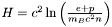, where e is the (total) energy density, p the pressure, n the baryon density, and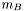the baryon mass nzet number of domains where the derivative dln(e)/dln(H) is to be computed. l_min index of the innermost domain is which the coefficient dln(n)/dln(H) is to be computed [default value: 0]; the derivative dln(e)/dln(H) is computed only in domains whose indices are in [l_min,l_min+nzet-1] . In the other domains, it is set to zero. par possible extra parameters of the EOS
Returns
dln(e)/dln(H)

Definition at line 454 of file eos.C.

## ◆ der_ener_ent() [2/2]

 Scalar Lorene::Eos::der_ener_ent ( const Scalar & ent, int nzet, int l_min = 0, Param * par = 0x0 ) const
inherited

Computes the logarithmic derivativefrom the log-enthalpy and extra parameters.

Parameters
 ent [input, unit:] log-enthalpy H defined by, where e is the (total) energy density, p the pressure, n the baryon density, andthe baryon mass nzet number of domains where the derivative dln(e)/dln(H) is to be computed. l_min index of the innermost domain is which the coefficient dln(n)/dln(H) is to be computed [default value: 0]; the derivative dln(e)/dln(H) is computed only in domains whose indices are in [l_min,l_min+nzet-1] . In the other domains, it is set to zero. par possible extra parameters of the EOS
Returns
dln(e)/dln(H)

Definition at line 464 of file eos.C.

## ◆ der_ener_ent_p()

 double Lorene::Eos_tabul::der_ener_ent_p ( double ent, const Param * par = 0x0 ) const
virtualinherited

Computes the logarithmic derivativefrom the log-enthalpy.

Parameters
 ent [input, unit:] log-enthalpy H
Returns
dln(e)/dln(H)

Implements Lorene::Eos.

Definition at line 523 of file eos_tabul.C.

## ◆ der_nbar_ent() [1/2]

 Cmp Lorene::Eos::der_nbar_ent ( const Cmp & ent, int nzet, int l_min = 0, Param * par = 0x0 ) const
inherited

Computes the logarithmic derivativefrom the log-enthalpy and extra parameters.

Parameters
 ent [input, unit:] log-enthalpy H defined by, where e is the (total) energy density, p the pressure, n the baryon density, andthe baryon mass nzet number of domains where the derivative dln(n)/dln(H) is to be computed. l_min index of the innermost domain is which the coefficient dln(n)/dln(H) is to be computed [default value: 0]; the derivative dln(n)/dln(H) is computed only in domains whose indices are in [l_min,l_min+nzet-1] . In the other domains, it is set to zero. par possible extra parameters of the EOS
Returns
dln(n)/dln(H)

Definition at line 431 of file eos.C.

## ◆ der_nbar_ent() [2/2]

 Scalar Lorene::Eos::der_nbar_ent ( const Scalar & ent, int nzet, int l_min = 0, Param * par = 0x0 ) const
inherited

Computes the logarithmic derivativefrom the log-enthalpy and extra parameters.

Parameters
 ent [input, unit:] log-enthalpy H defined by, where e is the (total) energy density, p the pressure, n the baryon density, andthe baryon mass nzet number of domains where the derivative dln(n)/dln(H) is to be computed. l_min index of the innermost domain is which the coefficient dln(n)/dln(H) is to be computed [default value: 0]; the derivative dln(n)/dln(H) is computed only in domains whose indices are in [l_min,l_min+nzet-1] . In the other domains, it is set to zero. par possible extra parameters of the EOS
Returns
dln(n)/dln(H)

Definition at line 441 of file eos.C.

## ◆ der_nbar_ent_p()

 double Lorene::Eos_tabul::der_nbar_ent_p ( double ent, const Param * par = 0x0 ) const
virtualinherited

Computes the logarithmic derivativefrom the log-enthalpy.

Parameters
 ent [input, unit:] log-enthalpy H
Returns
dln(n)/dln(H)

Implements Lorene::Eos.

Definition at line 500 of file eos_tabul.C.

## ◆ der_press_ent() [1/2]

 Cmp Lorene::Eos::der_press_ent ( const Cmp & ent, int nzet, int l_min = 0, Param * par = 0x0 ) const
inherited

Computes the logarithmic derivativefrom the log-enthalpy and extra parameters.

Parameters
 ent [input, unit:] log-enthalpy H defined by, where e is the (total) energy density, p the pressure, n the baryon density, andthe baryon mass nzet number of domains where the derivative dln(p)/dln(H) is to be computed. par possible extra parameters of the EOS l_min index of the innermost domain is which the coefficient dln(n)/dln(H) is to be computed [default value: 0]; the derivative dln(p)/dln(H) is computed only in domains whose indices are in [l_min,l_min+nzet-1] . In the other domains, it is set to zero.
Returns
dln(p)/dln(H)

Definition at line 476 of file eos.C.

## ◆ der_press_ent() [2/2]

 Scalar Lorene::Eos::der_press_ent ( const Scalar & ent, int nzet, int l_min = 0, Param * par = 0x0 ) const
inherited

Computes the logarithmic derivativefrom the log-enthalpy and extra parameters.

Parameters
 ent [input, unit:] log-enthalpy H defined by, where e is the (total) energy density, p the pressure, n the baryon density, andthe baryon mass nzet number of domains where the derivative dln(p)/dln(H) is to be computed. par possible extra parameters of the EOS l_min index of the innermost domain is which the coefficient dln(n)/dln(H) is to be computed [default value: 0]; the derivative dln(p)/dln(H) is computed only in domains whose indices are in [l_min,l_min+nzet-1] . In the other domains, it is set to zero.
Returns
dln(p)/dln(H)

Definition at line 486 of file eos.C.

## ◆ der_press_ent_p()

 double Lorene::Eos_tabul::der_press_ent_p ( double ent, const Param * par = 0x0 ) const
virtualinherited

Computes the logarithmic derivativefrom the log-enthalpy.

Parameters
 ent [input, unit:] log-enthalpy H
Returns
dln(p)/dln(H)

Implements Lorene::Eos.

Definition at line 543 of file eos_tabul.C.

## ◆ der_press_nbar_p()

 double Lorene::Eos_tabul::der_press_nbar_p ( double ent, const Param * par = 0x0 ) const
virtualinherited

Computes the logarithmic derivativefrom the log-enthalpy.

Parameters
 ent [input, unit:] log-enthalpy H
Returns
dln(p)/dln(n)

Definition at line 573 of file eos_tabul.C.

## ◆ ener_ent() [1/2]

 Cmp Lorene::Eos::ener_ent ( const Cmp & ent, int nzet, int l_min = 0, Param * par = 0x0 ) const
inherited

Computes the total energy density from the log-enthalpy and extra parameters.

Parameters
 ent [input, unit:] log-enthalpy H defined by, where e is the (total) energy density, p the pressure, n the baryon density, andthe baryon mass nzet number of domains where the energy density is to be computed. l_min index of the innermost domain is which the energy density is to be computed [default value: 0]; the energy density is computed only in domains whose indices are in [l_min,l_min+nzet-1] . In the other domains, it is set to zero. par possible extra parameters of the EOS
Returns
energy density [unit: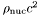], where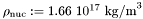Definition at line 387 of file eos.C.

References Lorene::Eos::calcule(), Lorene::Eos::ener_ent_p(), and Lorene::Cmp::get_mp().

## ◆ ener_ent() [2/2]

 Scalar Lorene::Eos::ener_ent ( const Scalar & ent, int nzet, int l_min = 0, Param * par = 0x0 ) const
inherited

Computes the total energy density from the log-enthalpy and extra parameters.

Parameters
 ent [input, unit:] log-enthalpy H defined by, where e is the (total) energy density, p the pressure, n the baryon density, andthe baryon mass nzet number of domains where the energy density is to be computed. l_min index of the innermost domain is which the energy density is to be computed [default value: 0]; the energy density is computed only in domains whose indices are in [l_min,l_min+nzet-1] . In the other domains, it is set to zero. par possible extra parameters of the EOS
Returns
energy density [unit:], whereDefinition at line 397 of file eos.C.

## ◆ ener_ent_p()

 double Lorene::Eos_tabul::ener_ent_p ( double ent, const Param * par = 0x0 ) const
virtualinherited

Computes the total energy density from the log-enthalpy.

Parameters
 ent [input, unit:] log-enthalpy H
Returns
energy density e [unit:], whereImplements Lorene::Eos.

Reimplemented in Lorene::Eos_consistent.

Definition at line 447 of file eos_tabul.C.

## ◆ eos_from_file() [1/2]

 Eos * Lorene::Eos::eos_from_file ( FILE * fich )
staticinherited

Construction of an EOS from a binary file.

The file must have been created by the function sauve(FILE*) .

Definition at line 193 of file eos_from_file.C.

## ◆ eos_from_file() [2/2]

 Eos * Lorene::Eos::eos_from_file ( ifstream & fich )
staticinherited

Construction of an EOS from a formatted file.

The fist line of the file must start by the EOS number, according to the following conventions:

• 1 = relativistic polytropic EOS (class Eos_poly ).
• 2 = Newtonian polytropic EOS (class Eos_poly_newt ).
• 3 = Relativistic incompressible EOS (class Eos_incomp ).
• 4 = Newtonian incompressible EOS (class Eos_incomp_newt ).
• 5 = Strange matter (MIT Bag model)
• 6 = Strange matter (MIT Bag model) with crust
• 10 = SLy4 (Douchin & Haensel 2001)
- 11 = FPS (Friedman-Pandharipande + Skyrme)
• 12 = BPAL12 (Bombaci et al. 1995)
• 13 = AkmalPR (Akmal, Pandharipande & Ravenhall 1998)
• 14 = BBB2 (Baldo, Bombaci & Burgio 1997)
• 15 = BalbN1H1 (Balberg 2000)
• 16 = GlendNH3 (Glendenning 1985, case 3)
• 17 = Tabulated EOS in CompOSE format
• 18 = magnetized (tabulated) equation of state
• 19 = relativistic ideal Fermi gas at zero temperature (class Eos_Fermi)
• 20 = Tabulated EOS in CompOSE format corrected for thermo. consistency
• 100 = Multi-domain EOS (class MEos )
• 110 = Multi-polytropic EOS (class Eos_multi_poly )
• 120 = Fitted SLy4 (Shibata 2004)
• 121 = Fitted FPS (Shibata 2004)
• 122 = Fitted AkmalPR (Taniguchi 2005)

The second line in the file should contain a name given by the user to the EOS. The following lines should contain the EOS parameters (one parameter per line), in the same order than in the class declaration.

Definition at line 345 of file eos_from_file.C.

## ◆ get_hmax()

 double Lorene::Eos_tabul::get_hmax ( ) const
inlineinherited

Returns maximal value of log-enthalpy in the table.

Definition at line 302 of file eos_tabul.h.

References Lorene::Eos_tabul::hmax.

## ◆ get_hmin()

 double Lorene::Eos_tabul::get_hmin ( ) const
inlineinherited

Returns minimal value of log-enthalpy in the table.

Definition at line 299 of file eos_tabul.h.

References Lorene::Eos_tabul::hmin.

## ◆ get_name()

 const char * Lorene::Eos::get_name ( ) const
inherited

Returns the EOS name.

Definition at line 179 of file eos.C.

References Lorene::Eos::name.

## ◆ identify()

 int Lorene::Eos_CompOSE::identify ( ) const
virtual

Returns a number to identify the sub-classe of Eos the object belongs to.

Implements Lorene::Eos.

Reimplemented in Lorene::Eos_consistent.

Definition at line 167 of file eos_from_file.C.

## ◆ nbar_ent() [1/2]

 Cmp Lorene::Eos::nbar_ent ( const Cmp & ent, int nzet, int l_min = 0, Param * par = 0x0 ) const
inherited

Computes the baryon density field from the log-enthalpy field and extra parameters.

Parameters
 ent [input, unit:] log-enthalpy H defined by, where e is the (total) energy density, p the pressure, n the baryon density, andthe baryon mass nzet number of domains where the baryon density is to be computed. l_min index of the innermost domain is which the baryon density is to be computed [default value: 0]; the baryon density is computed only in domains whose indices are in [l_min,l_min+nzet-1] . In the other domains, it is set to zero. par possible extra parameters of the EOS
Returns
baryon density [unit: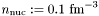]

Definition at line 362 of file eos.C.

References Lorene::Eos::calcule(), Lorene::Cmp::get_mp(), and Lorene::Eos::nbar_ent_p().

## ◆ nbar_ent() [2/2]

 Scalar Lorene::Eos::nbar_ent ( const Scalar & ent, int nzet, int l_min = 0, Param * par = 0x0 ) const
inherited

Computes the baryon density field from the log-enthalpy field and extra parameters.

Parameters
 ent [input, unit:] log-enthalpy H defined by, where e is the (total) energy density, p the pressure, n the baryon density, andthe baryon mass nzet number of domains where the baryon density is to be computed. l_min index of the innermost domain is which the baryon density is to be computed [default value: 0]; the baryon density is computed only in domains whose indices are in [l_min,l_min+nzet-1] . In the other domains, it is set to zero. par possible extra parameters of the EOS
Returns
baryon density [unit:]

Definition at line 372 of file eos.C.

## ◆ nbar_ent_p()

 double Lorene::Eos_tabul::nbar_ent_p ( double ent, const Param * par = 0x0 ) const
virtualinherited

Computes the baryon density from the log-enthalpy.

Parameters
 ent [input, unit:] log-enthalpy H
Returns
baryon density n [unit:]

Implements Lorene::Eos.

Reimplemented in Lorene::Eos_consistent.

Definition at line 419 of file eos_tabul.C.

## ◆ operator!=()

 bool Lorene::Eos_CompOSE::operator!= ( const Eos & eos_i ) const
virtual

Comparison operator (difference)

Implements Lorene::Eos.

Reimplemented in Lorene::Eos_consistent.

Definition at line 313 of file eos_compose.C.

References operator==().

## ◆ operator==()

 bool Lorene::Eos_CompOSE::operator== ( const Eos & eos_i ) const
virtual

Comparison operator (egality)

Implements Lorene::Eos.

Reimplemented in Lorene::Eos_consistent.

Definition at line 300 of file eos_compose.C.

References identify(), and Lorene::Eos::identify().

## ◆ operator>>()

 ostream & Lorene::Eos_CompOSE::operator>> ( ostream & ost ) const
protectedvirtual

Operator >>

Implements Lorene::Eos.

Reimplemented in Lorene::Eos_consistent.

Definition at line 330 of file eos_compose.C.

## ◆ press_ent() [1/2]

 Cmp Lorene::Eos::press_ent ( const Cmp & ent, int nzet, int l_min = 0, Param * par = 0x0 ) const
inherited

Computes the pressure from the log-enthalpy and extra parameters.

Parameters
 ent [input, unit:] log-enthalpy H defined by, where e is the (total) energy density, p the pressure, n the baryon density, andthe baryon mass nzet number of domains where the pressure is to be computed. l_min index of the innermost domain is which the pressure is to be computed [default value: 0]; the pressure is computed only in domains whose indices are in [l_min,l_min+nzet-1] . In the other domains, it is set to zero. par possible extra parameters of the EOS
Returns
pressure [unit:], whereDefinition at line 409 of file eos.C.

## ◆ press_ent() [2/2]

 Scalar Lorene::Eos::press_ent ( const Scalar & ent, int nzet, int l_min = 0, Param * par = 0x0 ) const
inherited

Computes the pressure from the log-enthalpy and extra parameters.

Parameters
 ent [input, unit:] log-enthalpy H defined by, where e is the (total) energy density, p the pressure, n the baryon density, andthe baryon mass nzet number of domains where the pressure is to be computed. l_min index of the innermost domain is which the pressure is to be computed [default value: 0]; the pressure is computed only in domains whose indices are in [l_min,l_min+nzet-1] . In the other domains, it is set to zero. par possible extra parameters of the EOS
Returns
pressure [unit:], whereDefinition at line 419 of file eos.C.

## ◆ press_ent_p()

 double Lorene::Eos_tabul::press_ent_p ( double ent, const Param * par = 0x0 ) const
virtualinherited

Computes the pressure from the log-enthalpy.

Parameters
 ent [input, unit:] log-enthalpy H
Returns
pressure p [unit:], whereImplements Lorene::Eos.

Reimplemented in Lorene::Eos_consistent.

Definition at line 475 of file eos_tabul.C.

protectedvirtual

Reads the files containing the table and initializes in the arrays logh , logp and dlpsdlh (CompOSE format).

Reimplemented in Lorene::Eos_consistent.

Definition at line 145 of file eos_compose.C.

protectedvirtualinherited

Reads the file containing the table and initializes in the arrays logh , logp and dlpsdlh .

Reimplemented in Lorene::Eos_consistent.

Definition at line 258 of file eos_tabul.C.

## ◆ sauve()

 void Lorene::Eos_CompOSE::sauve ( FILE * fich ) const
virtual

Save in a file.

Reimplemented from Lorene::Eos_tabul.

Definition at line 322 of file eos_compose.C.

References format, Lorene::fwrite_be(), and Lorene::Eos_tabul::sauve().

## ◆ set_name()

 void Lorene::Eos::set_name ( const char * name_i )
inherited

Sets the EOS name.

Definition at line 173 of file eos.C.

References Lorene::Eos::name.

## ◆ Eos::eos_from_file

 Eos* Eos::eos_from_file ( FILE * )
friend

The construction functions from a file.

## ◆ authors

 string Lorene::Eos_tabul::authors
protectedinherited

Authors - reference for the table.

Definition at line 194 of file eos_tabul.h.

## ◆ dlpsdlh

 Tbl* Lorene::Eos_tabul::dlpsdlh
protectedinherited

Table of.

Definition at line 209 of file eos_tabul.h.

## ◆ dlpsdlnb

 Tbl* Lorene::Eos_tabul::dlpsdlnb
protectedinherited

Table of.

Definition at line 215 of file eos_tabul.h.

## ◆ format

 int Lorene::Eos_CompOSE::format
protected

0 for standard (old) LORENE format, 1 for CompOSE format

Definition at line 99 of file eos_compose.h.

## ◆ hmax

 double Lorene::Eos_tabul::hmax
protectedinherited

Upper boundary of the enthalpy interval.

Definition at line 200 of file eos_tabul.h.

## ◆ hmin

 double Lorene::Eos_tabul::hmin
protectedinherited

Lower boundary of the enthalpy interval.

Definition at line 197 of file eos_tabul.h.

## ◆ log_cs2

 Tbl* Lorene::Eos_tabul::log_cs2
protectedinherited

Table of.

Definition at line 218 of file eos_tabul.h.

## ◆ logh

 Tbl* Lorene::Eos_tabul::logh
protectedinherited

Table of.

Definition at line 203 of file eos_tabul.h.

## ◆ lognb

 Tbl* Lorene::Eos_tabul::lognb
protectedinherited

Table of.

Definition at line 212 of file eos_tabul.h.

## ◆ logp

 Tbl* Lorene::Eos_tabul::logp
protectedinherited

Table of.

Definition at line 206 of file eos_tabul.h.

## ◆ name

 char Lorene::Eos::name
protectedinherited

EOS name.

Definition at line 212 of file eos.h.

## ◆ tablename

 string Lorene::Eos_tabul::tablename
protectedinherited

Name of the file containing the tabulated data.

Definition at line 192 of file eos_tabul.h.

The documentation for this class was generated from the following files: